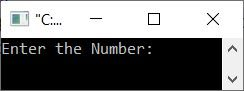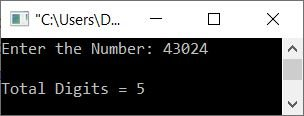# C++ Program to Count the Total Digits in a Number

This article provides some programs in C++ that count the total number of digits available in a number entered by the user at run-time of the program. This article deals with:

For example, if the user enters a number like 13204, the output will be 5. because there are 5 digits available in the given number.

## Using the while loop, count the total digits in a number

The question is: write a C++ program that receives a number from the user and counts and prints the total number of digits available in that given number. Here is the answer to this question:

```#include<iostream>

using namespace std;
int main()
{
int num, tot=0;
cout<<"Enter the Number: ";
cin>>num;
while(num>0)
{
tot++;
num = num/10;
}
cout<<"\nTotal Digits = "<<tot;
cout<<endl;
return 0;
}```

Here is the initial output produced by the above C++ program on counting the total number of digits available in a given number by the user at run-time:Now enter a number, say 43024, as input and press the ENTER key to see the output as shown in the snapshot given below:The following is the dry run of the above program with user input 43024:

• When the user enters the number, it gets stored in the "num" variable. So num=43024.
• After receiving the number from the user, the execution of the "while" loop begins.
• That is, the condition num>0 or 43024>0 is satisfied.
• Therefore, program flow goes inside the loop.
• The value of "tot" gets incremented, because the initial value of tot is 0. Therefore, tot = 1 now.
• The statement num = num/10; is then executed.
• Therefore, num/10 or 43024/10 or 4302 gets initialized to "num". So num=4302
• Again, the condition of the "while" loop gets evaluated.
• In other words, the condition num>0 or 4302>0 evaluates to true.
• Therefore, program flow again goes inside the loop.
• The value of "tot" again gets incremented. So tot = 2 now.
• And num/10 or 4302/10 or 430 is set to num. As a result, n = 430 now.
• Again, the third time, the condition of the "while" loop gets evaluated.
• This process continues until the condition is evaluated as false.
• In this way, after exiting from the loop, or when the condition evaluates to be false. The variable "tot" holds a value that is equal to the total number of digits available in the number.
• Therefore, just print the value of "tot" as output.

## Count Digits in a Number using the for Loop

This program does the same job as the previous program. The only difference is its approach. That is, unlike the previous program, this one is written in a for loop rather than a while loop.

```#include<iostream>

using namespace std;
int main()
{
int num, tot;
cout<<"Enter the Number: ";
cin>>num;
for(tot=0; num>0; tot++)
num = num/10;
cout<<"\nTotal Digits = "<<tot;
cout<<endl;
return 0;
}```

This program produces the same output as the previous program.

## Using a Function, Count the Digits in a Number

This is the last program on counting the total number of digits available in a number entered by the user using a user-defined function like shown in the program given below:

```#include<iostream>

using namespace std;

int myfun(int);
int main()
{
int num, tot;
cout<<"Enter the Number: ";
cin>>num;
tot = myfun(num);
cout<<"\nTotal Digits = "<<tot;
cout<<endl;
return 0;
}
int myfun(int n)
{
int t;
for(t=0; n>0; t++)
n /= 10;
return t;
}```

C++ Quiz

« Previous Program Next Program »